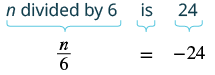## Translating Words Involving Division and Multiplication Into an Algebraic Equation and Solving

### Learning Outcomes

• Translate a phrase that contains division into an equation and solve

## Translate Sentences to Equations and Solve

Recall the four properties of equality—subtraction, addition, division, and multiplication. We’ll list them all together here for easy reference. We will use these to solve equations that contain fractions.

 Subtraction Property of Equality: For any real numbers $\mathit{\text{a, b,}}$ and $\mathit{\text{c,}}$ if $a=b$, then $a-c=b-c$. Addition Property of Equality: For any real numbers $\mathit{\text{a, b,}}$ and $\mathit{\text{c,}}$ if $a=b$, then $a+c=b+c$. Division Property of Equality: For any numbers $\mathit{\text{a, b,}}$ and $\mathit{\text{c,}}$ where $\mathit{\text{c}}\ne \mathit{0}$ if $a=b$, then $\Large\frac{a}{c}= \Large\frac{b}{c}$ Multiplication Property of Equality: For any real numbers $\mathit{\text{a, b,}}$ and $\mathit{\text{c}}$ if $a=b$, then $ac=bc$

When you add, subtract, multiply or divide the same quantity from both sides of an equation, you still have equality.
In the next few examples, we’ll translate sentences that contain fractions into equations and then solve the equations. The first property of equality we will use is multiplication.

### Example

Translate and solve: $n$ divided by $6$ is $-24$.

Solution:

 Translate.Multiply both sides by $6$ . $\color{red}{6}\cdot\Large\frac{n}{6}\normalsize=\color{red}{6}(-24)$ Simplify. $n=-144$ Check: Is $-144$ divided by $6$ equal to $-24$ ? Translate. $\Large\frac{-144}{6}\normalsize\stackrel{?}{=}-24$ Simplify. It checks. $-24=-24\quad\checkmark$

### Example

Translate and solve: The quotient of $q$ and $-5$ is $70$.

### Example

Translate and solve: Two-thirds of $f$ is $18$.

### Example

Translate and solve: The quotient of $m$ and $\Large\frac{5}{6}$ is $\Large\frac{3}{4}$.

### Example

Translate and solve: The sum of three-eighths and $x$ is three and one-half.

### try It

We’ve seen several examples of how to translate a given mathematical relationship from words into equation form in order to solve it. Let’s see some examples now that reverse the process. The following video shows examples of translating an equation into words as an aid to solving it. Note that this is different from the written examples on this page because these examples start with the mathematical equation then translate it into words.